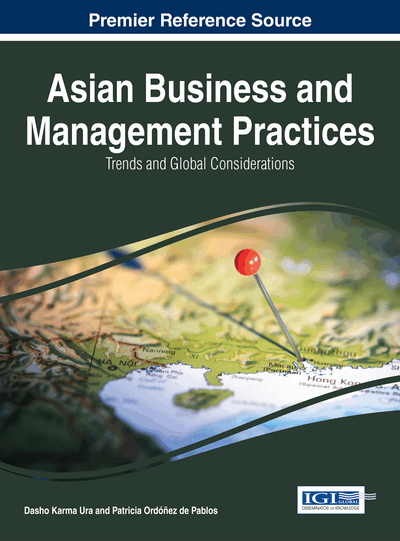# An Importance Sampling Method for Expectation of Portfolio Credit Risk

Yue Qiu (Capital University of Economics and Business, China) and Chuansheng Wang (Capital University of Economics and Business, China)
DOI: 10.4018/978-1-4666-6441-8.ch016
Available
\$37.50
No Current Special Offers

## Abstract

Simulation is widely used to estimate losses due to default and other credit events in financial portfolios. The accurate measurement of credit risk can be modeled as a rare event simulation problem. While Monte Carlo simulation is time-consuming for rare events, importance sampling techniques can effectively reduce the simulation time, thus improving simulation efficiency. This chapter proposes a new importance sampling method to estimate rare event probability in simulation models. The optimal importance sampling distributions are derived in terms of expectation in the normal copula model developed in finance. In the normal copula model, dependency is introduced through a set of common factors of multiple obligors. The intriguing dependence between defaults of multiple obligors imposes hurdles in simulation. The simulated results demonstrate the effectiveness of the proposed approach to solving the portfolio credit risk problem.
Chapter Preview
Top

## 1. Introduction

Sequence Given a credit risk model, the rapid and accurate construction of the portfolio loss distribution is at the heart of credit risk management. This is particularly true for accurate estimation of small but important probabilities of large losses, which are usually the focus of risk measurement. Monte Carlo simulation is frequently used to estimate this distribution. For high-quality portfolios, the accurate measurement of credit risk is often regarded as a rare event simulation problem. In this paper, the normal copula model is investigated, which originally associated with J.P. Morgan’s CreditMetrics system and now widely used (Gupton, Finger, & Bhatia 1997). We analyze the mathematical structure of portfolio credit risk models with particular regard to the modeling of dependence between default events in these models.

Rare event simulation has attracted extensive attentions since the concept was first proposed, but there haven’t been many research results yet. Straightforward simulation for rare events requires a large number of trials and hard to implement because the occurrence of rare events are extremely little in a standard simulation, hence new methods are needed to be investigated and developed. A more efficient simulation needs variance reduction techniques such as importance sampling (IS) (Siegmund, 1976; Devetsikiotis & Townsend, 1993). The main idea of IS is to make the occurrence of rare events more frequent by carrying out the simulation with a different probability distribution – what is the so-called change of measure (CM) – and to estimate the probability of interest via a corresponding likelihood ratio (LR) estimator. It is commonly known that the greatest difficulty in obtaining an efficient IS method is to find out the optimal importance sampling distribution function. It has been shown that the computation and experiments required for achieving an efficient IS method may be even more complicated than the original problem. Thus, this implies that we have to find simple and efficient IS algorithms. There are two main ways to solve this problem. One is to utilize Variance Minimization (VM) technique; the other is to use minimizing Cross Entropy (CE) technique. It is well known that there theoretically exists a CM that yields a zero-variance likelihood ratio estimator . However, in practice such an optimal CM cannot be computed since it depends on the underlying quantity being estimated. However, investigations have shown that the reduced variance method can be employed to approach the optimal solution in the sense of minimizing the variance. In other words, minimizing estimator's variance with original density function is equivalent to minimizing the expected likelihood ratio conditioned on the rare event happening; and minimizing cross entropy is equivalent to minimizing the expected logarithm of likelihood ratio conditioned on the rare event happening. Therefore, minimizing cross entropy in a sense is close to, but definitely is different from minimizing estimator's variance. In terms of estimator's variance, cross entropy method does not seek for the optimal solution. The cross entropy method is a powerful technique to compute the probabilities of rare events, which was first introduced by Rubinstein for rare event simulation and later for combinatorial optimization in Rubinstein (Rubinstein, 1997, 1999, 2001). Margolin and Costa et al. presented theoretical convergence results on the cross-entropy method (Margolin, 2005; Costa, Jones, & Kroese, 2007). The most important features of CE have been thoroughly exposed in de Boer et al (De Boer, Kroese, & Mannor, 2005). It has attracted the attentions of investigators from various research areas since it was proposed, such as vehicle routing (Chepuri & Homem-de-Mello, 2005), the max-cut problem (Rubinstein, 2002; Laguna, Duarte, & Martí, 2009), buffer allocation (Alon, Kroese, & Raviv, 2005), the integer knapsack problem (Caserta, Quiñonez, & Márquez, 2008), the multi-item multi-period capacitated lot-sizing problem (Caserta & Quiñonez, 2009). The complex dependence between defaults of multiple obligors complicates the application of IS. Glasserman and Li provided an IS procedure for the normal copula model, which employed a two-phase procedure in order to deal with the dependency (Glasserman & Li, 2003, 2005). However, it is hard to obtain the importance sampling density function; hence they had to use an approximation procedure.

## Complete Chapter List

Search this Book:
Reset